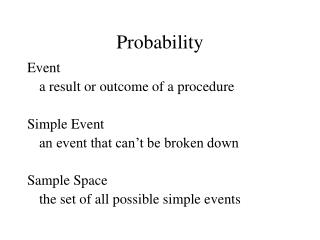DownloadDownload PresentationProbability

Probability

Télécharger la présentationProbability

- - - - - - - - - - - - - - - - - - - - - - - - - - - E N D - - - - - - - - - - - - - - - - - - - - - - - - - - -
Presentation Transcript

1. Probability Event a result or outcome of a procedure Simple Event an event that can’t be broken down Sample Space the set of all possible simple events

2. Example: you plan to have 3 kids Event 2 are girls Simple Event 1st is boy, 2nd and 3rd are girls (BGG) Sample Space {BBB,BBG,BGB,GBB,BGG,GBG,GGB,GGG}

3. Three approaches to probabilty Relative Frequency Approximation (Rule 1) Determine probability based on observed outcomes number of times A occurred P(A) = number of trials

4. Three approaches to probabilty Classical Approach (Rule 2) When all possible simple events have equal likelihood, number of ways A can occur P(A) = number of different simple events

5. Three approaches to probabilty Subjective Probabilities (Rule 3) Making an educated guess

6. Some probabilities Out of 300 people sampled, 165 said they preferred dogs over cats. What is the probability that a person chosen at random prefers dogs over cats? P(prefers dogs) =

7. Some probabilities Out of a group sampled, 165 said they preferred dogs, 120 said they preferred cats, and 15 liked both equally. What is the probability that a person chosen at random prefers dogs? P(prefers dogs) =

8. Some probabilities If you plan to have 3 kids, what’s the probability of exactly 1 girl? Sample space: BBB,BBG,BGB,GBB,BGG,GBG,GGB,GGG} P(1 girl) =

9. Some probabilities What’s the probability that you’ll trip and fall on your way back to your car?

10. Law of Large Numbers The relative frequency probability tends to approach the actual probability the more times the procedure is repeated In other words, the more observations we have to work from, the more accurate our probability will be.

11. Fundamental facts The probability of an impossible event is 0 The probability of a event that definitely will occur is 1 All probability values are between 0 and 1.

12. Complement The complement of an event A (denoted ) is the set of all events in which A does not occur. Example: A = get heads on coin flip = get tails on coin flip

13. Homework 3.2 4, 5, 7, 9, 15, 19